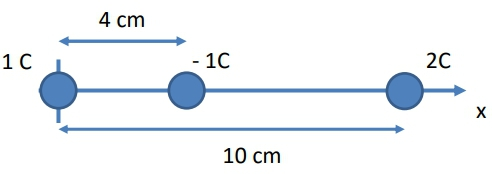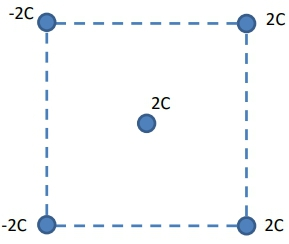## Physics

Learn the toughest concepts covered in Physics with step-by-step video tutorials and practice problems by world-class tutors

24. Electric Force & Field; Gauss' Law

# Coulomb's Law (Electric Force)

1
concept

## Coulomb's Law9m
Play a video:
2
Problem

If the force between two charges is F when the distance is d, what will the force between the two charges be if they were moved to a distance of 2d?

3
example

## Charges In A Line (Find Zero Force)7m
Play a video:
4
Problem

In which direction will the −1 C charge move? If it has a mass of 10 g, what will its initial acceleration be?5
example

## Charges In A Triangle (Rank Force Pairs)1m
Play a video:
Hey, guys. So in this problem, we have to rank although the possible pairs of charges in this diagram by which one has the greatest possible electric force. So we're talking about cool owns law and specifically for point charges. So we've got to use columns. Law K Q one Q two divided by the distance between them square. That's little are in this diagram, though we actually have the distance as D, but it's the same exact distance through all of the points in this triangle, which means that this D isn't gonna be a factor in determining which one is the strongest in which one is the weakest. Instead, what we have to dio is we have to just look at the product of the charges themselves, and we have three pairs that we're gonna discuss. We're going to discuss the pair between two and three e to e and sorry to an e two and three and then e and three. So I've got these little loops right here that represent those pears, and all we have to do is just compare the magnitude of the charges involved. Now, all these air like charges that all exert repulsive forces on each other, so we just have to figure what Q one Q two is Now. I've got to e and E and remember that e stands for the elementary charge, so I've got to eat times E. So, in other words, that's just gonna be two e squared. Remember that E is just 1.6 times 10 to the minus 19 just stands for, like, the electron or the country charge. And if I do the same exact thing over here, I've got Q one. Q two is equal to two e times three. And then that's just gonna equal six e squared. And then I've got Zoo Wannabe, the minus sign and then for the last one. I've got e times three. So that's just gonna be three e squared, right? That's Q one Q. Two. All right, so we're just gonna basically plug in these things if we're actually trying to figure out what the forces are inside for Q one. Q. Two, Which one is the greatest? Well, the six e squared is first, and then the three e squared is next. And then, finally, this two e squared is third, so that's That's the rank in terms off, sort of descending, greatest electric force. Alright, guys, let me know if you guys have any questions with that.
6
example

## Charges in a Plane7m
Play a video:
7
example

## Exploiting Symmetry3m
Play a video:
Hey, guys. So in this video, we're gonna cover something really, really important That's gonna help you in a lot of problems called exploiting symmetry. And it happens when you have these sort of arrangements of charges. Let's go ahead and check out two examples. So in this example, we've got to to cool, um, charges, stationed away from this one Coolum charge. And we want to figure out just the direction, not the magnitude off the net force on this thing. All right, so let's go ahead and work out what the forces acting on that are on the one column charge. There's a repulsive force coming from the two column charge and there's also repulsive force due to the other two Coolum charge. Now, if you wanted to figure out what the net force is, we have to calculate those forces and then decompose them. Right, So we've got f here and then we've got f over here. Alright, so we wanna exploit something called symmetry. Notice how these two Coolum charges are both position the same distance away, so usually have symmetry. Whenever you have the same cues, the same charges positioned in the same distance away So you have distance or are it could be whatever letter they choose to be the distance. Now, if you have these two things, the same charge and you have the same distance that means that the force acting on these forces, both of these efs here are also the same. So in order to figure out the net force would have to decompose them into their components. Right? So we have an ex components and we have a white components over here and we do that by using our trig are signs and co signs and things like that if you could figure out what that angle is, but we also have to do the same thing over here there's an ex components, there is a angle theta and there is a white component. So what happens is if you have the same efs and these things are symmetrically placed, then you're gonna have the same exact angle feta. And in this situation, what's gonna happen is that the opposite components here are gonna have the same exact magnitude. So these things are always going to cancel. You'll never have to worry about these X components and the white components are basically going to add together, and they'll be the exact same thing. Whoa! So they're always going to add together. And so that means the net force of this object is not just going to be somewhere pointing upwards. It's going to be exactly pointing upwards. The net force is just going to be the sum of both of the white components here. Alright, so that's one week where you can use symmetry. Let's see another example. So in this situation, it's gonna be very similar, except instead of both of them being positive charges, we have one positive and one negative. Okay, so we know that there's a repulsive force from this positive to cool, um, charge. But now this negative to cool. Um, charge over here is gonna exert a attractive force because these things are unlike charges, so we're gonna have a attractive force that points off in this direction. Now, these are gonna have these are gonna be f and f over here. We've got the same exact distance and the same exact charges. So that means these forces were gonna be the same. So we've got same cues. We've got the same distance so that means the forces were gonna be the same. And these things are position, sort of like in a symmetrical way, which means we're gonna have the same exact data. So means when we start breaking these things up into their components, right, we've got the white components, the X components by using that trig well, the attractive force this red one can also be broken up into its components. And we're gonna have the same exact angle theta. So it's gonna end up happening is we've satisfied all the conditions for symmetry. So that means that the components in the Y direction, the ones that point in opposite directions are always gonna cancel out. And now the Net force over here is going to be in the purely X directions things. Net force is going to be two times three x component off both of those forces together. All right, so this is just the direction. So this is a really, really important thing they were gonna use. See if you could exploit some symmetry in your problems. Sometimes you'll give him like squares with a whole bunch of charges. You'll have to figure what the directions of the Net force. It's very important. Shortcut. You guys need to know. Let me know if you have any questions.
8
Problem

What is the direction of the net force on the charge at the center of the square in the following figure?9
example

## Electroscope (Find Charge)7m
Play a video: